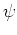Previous: 6.1.3 The Riccati and Up: 6. Mathematical Formulations of Next: 6.2.1 Phase Space Distribution

# 6.2 Quantum Mechanics in Phase Space

Formally, the phase space representation is closer to its classical counterpart than Schrödinger's formulation. The formulation of quantum mechanics in phase space is outside the scope of most introductory books on quantum mechanics. Here we present an introduction, which focuses on the Wigner distribution.

First we translate the abstract quantum mechanical machinery (operators and probabilities) to the Wigner language. Special care is devoted to the notion of marginal distributions and marginal expectation values. We point out that these present an instance of the operator ordering problem and their definition is non-unique. We then present a phase space distribution which has the property that it is positive everywhere and gets carrier, current and energy density consistent with the standard definitions for a Schrödinger wave function. Finally we discuss the quantum mechanical analogon of the classical notion of trajectories and their peculiarities.

SubsectionsPrevious: 6.1.3 The Riccati and Up: 6. Mathematical Formulations of Next: 6.2.1 Phase Space Distribution